#Quality Digest

Featured Product
This Week in Quality Digest Live
Six Sigma Features
Douglas C. Fair
Part 3 of our series on SPC in a digital era
Scott A. Hindle
Part 2 of our series on SPC in a digital era
Donald J. Wheeler
Part 2: By trying to do better, we can make things worse
Douglas C. Fair
Introducing our series on SPC in a digital era
Donald J. Wheeler
Part 1: Process-hyphen-control illustrated
Six Sigma News
How to use Minitab statistical functions to improve business processes
Sept. 28–29, 2022, at the MassMutual Center in Springfield, MA
Elsmar Cove is a leading forum for quality and standards compliance
Is the future of quality management actually business management?
Too often process enhancements occur in silos where there is little positive impact on the big picture
Collect measurements, visual defect information, simple Go/No-Go situations from any online device
Good quality is adding an average of 11 percent to organizations’ revenue growth
Floor symbols and decals create a SMART floor environment, adding visual organization to any environment
A guide for practitioners and managersBio

Six Sigma

## Don’t We Need to Remove the Outliers?

### Characterization and estimation are different

Published: Monday, October 6, 2014 - 09:56

Much of modern statistics is concerned with creating models which contain parameters that need to be estimated. In many cases these estimates can be severely affected by unusual or extreme values in the data. For this reason students are often taught to polish up the data by removing the outliers. Last month we looked at a popular test for outliers. In this column we shall look at the difference between estimating parameters and characterizing process behavior.

### Estimation

To illustrate how polishing the data can improve our estimates, we will use the data in figure 1. These values are 100 determinations of the weight of a 10-gram chrome steel standard known as NB10. These values were obtained once each week at the Bureau of Standards, by one of two individuals, using the same instrument each time. The weights were recorded to the nearest microgram. Because each value has the form of 9,999,xxx micrograms, the four nines at the start of each value are not shown in the table—only the last three values in the xxx positions are recorded. The values are in time order by column.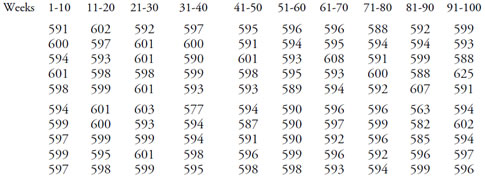Figure 1: NB10 values for weeks 1 to 100

If we compute the usual descriptive statistics, we find that the average of the tabled values is 595.4 micrograms, and their standard deviation statistic is 6.47 micrograms. Using these two values to define a normal distribution, we would end up with the curve shown superimposed upon the histogram in figure 2. Both the area under the curve and the area of the histogram are the same, yet the curve does not really match up with the histogram. It is too heavy in the regions around 585 and 605, and not high enough near 595.Figure 2: Histogram and normal curve for NB10 values

The outliers in the histogram create the mismatch between the fitted model and the data. Seven values look like outliers in figure 2. If we delete the four values below 586 and the three values above 606, and recompute our descriptive statistics, we find the revised histogram has an average of 595.6 micrograms and a standard deviation statistic of 3.74 micrograms. Using these two values to define a normal distribution, we end up with the curve shown in figure 3. Now we have a much better fit between our model and the histogram.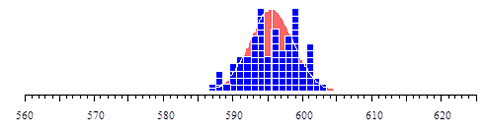Figure 3: Histogram and normal curve for revised NB10 values

The whole operation of deleting outliers to obtain a better fit between the model and the data is based upon computations which implicitly assume that the data are homogeneous. However, when you have outliers, this assumption becomes questionable. If the data are homogeneous, where did the outliers come from? Thus, whether the data are homogeneous or not must be the primary question for any type of analysis. Although this is the one question we do not address in our statistics classes, it is precisely the question considered by the process behavior chart.

### The characterization of process behavior

What about the seven values we simply deleted to obtain the better fit between our assumed model and our revised data set? What were these values trying to tell us about this process? Here the question is not one of estimation, but rather one of using the data to characterize the underlying process represented by the data.

Figure 4 contains the XmR chart for the 100 values of figure 1. The limits are based upon the median moving range of 4.0 micrograms. Here we have clear evidence of at least three upsets or changes in the process of weighing NB10. Five of the seven outliers that we deleted to fit the model in figure 3 are signals that reveal that this set of values is not homogeneous. This lack of homogeneity undermines the model of figure 3 and makes it inappropriate. If you want to use your data to gain insight into the underlying process that creates the data, then the outliers are the most important values in the data set! However, students are routinely taught to delete those pesky outliers. After all, when you are looking for iron and tin, you should not let silver and gold get in the way.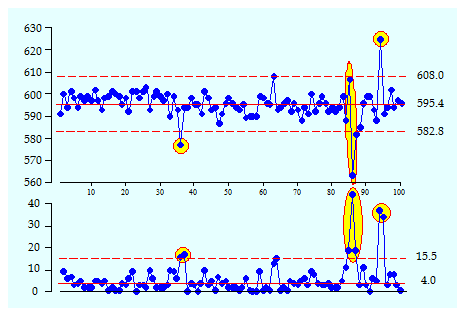Figure 4: XmR chart for 100 weighings of NB10

### Don’t the outliers distort the limits?

But don’t we need to remove the outliers to compute good estimates of location and dispersion? No, we don’t. To see why this is so, it is helpful to consider the effect of outliers upon the limits of a process behavior chart.

We commonly base our limits on the average and an average range. The average may be affected by some very extreme values, but this effect is usually much smaller than people think it will be. In figure 1 some values are out of line with the bulk of the data by as much as 30 micrograms. However, the average value of approximately 595 micrograms was found by dividing 59,500 by 100. If the total of 59,500 is adjusted up or down by 30, 60, or even 90 units, it will have a very small effect upon the average. In this example deleting the outliers changed the average from 595.4 to 595.6. Thus, the average is a very robust measure of location, which is why we use it as our main statistic for location. Of course, whenever we have reason to think that the average may have been affected by the presence of several extreme values that are all on the same side, we can always use the median instead. Hence, while our primary measure of location is robust, we have an alternative for those cases where one is needed.

Likewise, when we compute an average range, we are once again diluting the effect of any extreme values that are present in the set of ranges. In general, a few large ranges will not have an undue effect upon the average range. However, if they do appear to have inflated the average range, we can resort to using the median range. In figure 4 the limits are based upon the median moving range of 4.0 micrograms. This results in an estimated dispersion for the individual values of: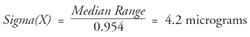It is instructive to compare this with the two values for the standard deviation statistic computed from these data. Using all 100 values from figure 1, we found s = 6.47 micrograms. Using only the 93 values shown in figure 3, we found s = 3.74 micrograms. Thus, the median moving range (based on all 100 values) gives an estimate for dispersion that is quite similar to the descriptive statistic computed after the outliers had been removed. This robustness that is built into the computations for the process behavior charts removes the need to polish the data prior to computing the limits. The computations work even in the presence of outliers and signals of exceptional variation.

### Don’t we need a predictable range chart?

The fact that the computations work even in the presence of outliers is important in light of the advice given in some SPC texts. These texts warn the student to check the range chart before computing limits for the X chart or the average chart. If the range chart is found to display evidence of unpredictable behavior, then the student is advised to avoid computing limits for the average chart or the X chart, the idea being that signals on the range chart will corrupt the average range and hence corrupt the limits on the other chart. This advice is motivated by a desire to avoid using anything less than the best estimates possible. However, the objective of a process behavior chart is not to estimate, but rather to characterize the process as being either predictable or unpredictable.

Given the conservative nature of three-sigma limits, we do not need high precision in our computations. Three-sigma limits are so conservative that any uncertainty in where our computed limits fall will not greatly affect the coverage of the limits. To characterize this effect, figure 5 shows the coverages associated with limits ranging from 2.8 sigma to 3.2 sigma on either side of the mean. There we see that regardless of the shape of the distribution, and regardless of the fact that there is uncertainty in our computations, our three-sigma limits are going to filter out virtually all of the routine variation. This is what allows us to compute limits and characterize process behavior without having to first delete the outliers. The computations are robust, and as a consequence, the technique is sensitive.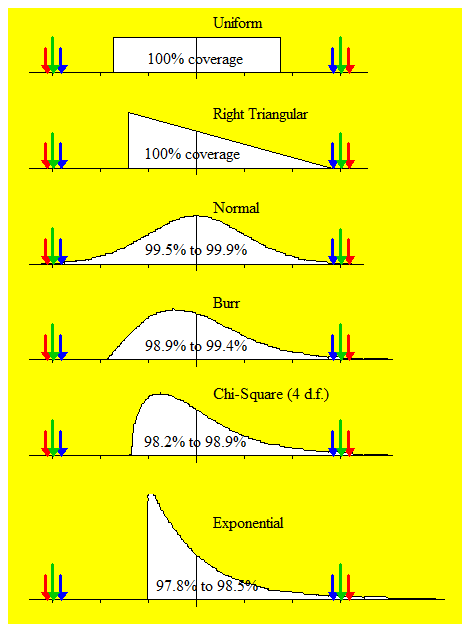Figure 5: Three-sigma limits filter out virtually all of the routine variation regardless of the shape of the histogram and regardless of the uncertainty in our estimates of the limits.

Thus, the advice to make sure the range chart is predictable prior to computing limits for the average chart or the X chart is just another version of the “delete the outliers” argument. These arguments are built on a misunderstanding of the objective of process behavior charts and a failure to appreciate that the computations are already robust.

### Summary

So, should you delete outliers before you place your data on a process behavior chart? Only if you want to throw away the most interesting and valuable part of your data!

If you fail to identify the signals of exceptional variation as such, if you assume that a collection of data is homogeneous when it is not, then you are likely to have both your analysis and your conclusions undermined. The outliers are the interesting part of your data. In the words of George Box, “The key to discovery is to get alignment between an interesting event and an interested observer.” Outliers tell you where to look to learn something about the underlying process that is generating your data. When you delete your outliers, you are losing an opportunity for discovery.

### Discuss### Donald J. Wheeler

Dr. Wheeler is a fellow of both the American Statistical Association and the American Society for Quality who has taught more than 1,000 seminars in 17 countries on six continents. He welcomes your questions; you can contact him at djwheeler@spcpress.com.

### Fantastic as always ... not

Fantastic as always ... not that most folk will pay any attention ...

### An alternative path that might be as bad as deleting the outlier

For the 100 data found in this article a method I observed for calculating what I have found described as a robust GLOBAL SD gives a value of 4.77 (i.e. no data deleted) (My point is not to dwell on the formula but instead to focus on the logic, or pevalence, of using methods of computing SD that pay no attention to time order of the data)

Here my question: In other organisations/industries is it common, when dealing with process data, to find summary statistics of dispersion computed using such GLOBAL measures that are described as robust?

As already mentioned, by being GLOBAL, such measures pay no attention to the observational order of the data (unlike average/median measures of dispersion used for the process behaviour chart).

What is interesting here is that:

- using the so-called robust (global) SD, the value of 4.77 takes us closer to the "good model", and this computational method giving us 4.77 is demonstrably less affected by the outliers (so one could proceed mathematically without deleting them)

- but, and probably most importantly, since we haven't addressed "why" the outliers occurred we leave ourselves open to suffer the consequences of their effect in the future (e.g. rework/scrap/ unhappy customers...)

So, I propose that, with process data, a robust GLOBAL SD is just another way of going down the wrong path. This path may help us to get closer to a "good model" (without deleting the outliers) but, by failing to address and deal with the cause/s behind the outliers, we leave ourselves open to a greater risk of less consistent quality in the future which could end up being scrap or rework ...

Scott.